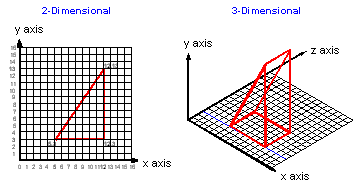# x-y matrix

Also found in: Dictionary, Thesaurus, Medical, Financial.

## x-y matrix

A group of rows and columns. The x-axis is the horizontal row, and the y-axis is the vertical column. An x-y matrix is the reference framework for two-dimensional structures, such as mathematical tables, display screens, digitizer tablets, dot matrix printers and 2D graphics images.Site: Follow: Share:
Open / Close